• 序言鉴于现在飞控的热度不减当年，越来越多“年轻人”加入飞控制作学习的阶段，也有许多新手上来就气势汹汹的进行姿态解算，往往使用的就是较为常用的Mahony的互补滤波姿态解算，笔者在多年前也一样，最开始接触的...
序言

鉴于现在飞控的热度不减当年，越来越多“年轻人”加入飞控制作学习的阶段，也有许多新手上来就气势汹汹的进行姿态解算，往往使用的就是较为常用的Mahony的互补滤波姿态解算，笔者在多年前也一样，最开始接触的就是这个算法，但是当时懂得马马虎虎，直接把数据丢进去之后就得到了解算好的四元数，之后按照网上的公式一转换就OK了，但是后来才发现，当你的飞控出现问题的时候，大多数的时候，不见得是控制问题，有时候确实是因为姿态解算不好，所以今天就特别的对这个算法进行讲解，引用一个最近看到的：我希望这篇博客可以帮助到一些人。

姿态解算的基本概念

说是概念，其实确切来讲是应该弄清楚的事情：
1、姿态解算中的传感器的特性。对于加速度，其大小使用三角公式得到一个角度（笔者这么干过，确实行之有效），但是这个角度在旋转瞬间是十分不稳定的，例如在水平面转到10度左右的时候，这个角度很可能超过20度，之后会慢慢回到10度，即所谓的“短期不稳定，长期稳定”；对于角速度，可以通过“角度=∫角速度dt”这样的公式得到角度，但是这样做的时候会发现当在瞬间旋转的时候，得到的角度差是准确的，但是一旦长期不矫正这个角度，这个角度就会远远的偏离实际的角度，即所谓的“短期稳定，长期不稳定”；而磁力计的特性就偏好，短期和长期都可以很稳定，但是在飞机倾斜的时候，原本的磁场数据就会变得不准确，需要校准。
2、姿态解算中谁是主角。在接触飞控之初，我觉得加速度应该是主力（当时还不知道yaw轴长期的漂移现象），毕竟他的数据是长期稳定的，但是很遗憾，在当下接触到的姿态解算算法，大多数是以角速度作为姿态解算的主角，这点希望初学者要谨记在心。
3、DCM矩阵（方向余弦矩阵），这个名词对于飞控的“高阶玩家”确实不应该感到陌生，但是对于初学者，可能知道更多的是四元数，但是笔者在这里还是要说一下，在姿态解算中，甚至到后面的定高、定点算法中，DCM矩阵都会起到很大的作用，而不是四元数。简单的来讲，DCM矩阵的作用就是把A坐标系的数据旋转到B坐标，举个简单的例子，你可以通过DCM矩阵把机体上的三轴加速度计数据旋转到世界坐标系下。

姿态解算算法介绍

void IMUupdate(float gx, float gy, float gz, float ax,float ay, float az)
{
float norm;
float vx, vy, vz;
float ex, ey, ez;

float q0q0 = q0*q0;
float q0q1 = q0*q1;
float q0q2 = q0*q2;
float q1q1 = q1*q1;
float q1q3 = q1*q3;
float q2q2 = q2*q2;
float q2q3 = q2*q3;
float q3q3 = q3*q3;

if(ax*ay*az==0)
return;

// 第一步：对加速度数据进行归一化
norm = sqrt(ax*ax + ay*ay + az*az);
ax = ax / norm;
ay = ay / norm;
az = az / norm;

// 第二步：DCM矩阵旋转
vx = 2*(q1q3 - q0q2);
vy = 2*(q0q1 + q2q3);
vz = q0q0 - q1q1 - q2q2 + q3q3 ;

// 第三步：在机体坐标系下做向量叉积得到补偿数据
ex = ay*vz - az*vy ;
ey = az*vx - ax*vz ;
ez = ax*vy - ay*vx ;

// 第四步：对误差进行PI计算，补偿角速度
exInt = exInt + ex * Ki;
eyInt = eyInt + ey * Ki;
ezInt = ezInt + ez * Ki;

gx = gx + Kp*ex + exInt;
gy = gy + Kp*ey + eyInt;
gz = gz + Kp*ez + ezInt;

// 第五步：按照四元数微分公式进行四元数更新
q0 = q0 + (-q1*gx - q2*gy - q3*gz)*halfT;
q1 = q1 + (q0*gx + q2*gz - q3*gy)*halfT;
q2 = q2 + (q0*gy - q1*gz + q3*gx)*halfT;
q3 = q3 + (q0*gz + q1*gy - q2*gx)*halfT;
}


以上就是网上比较流行的姿态解算程序，在此笔者把最精华的部分截取出来进行大概的讲解，在笔者心里，这个算法主要分为五个步骤：
1、对数据的规范化（注意，这里是规范化，程序注解是归一化），什么意思，就是对于姿态解算来讲，数据是要在使用这个算法之前进行标度变换的！首先是角速度，大部分的传感器输出的角速度是“度每秒”，但是实际上，角速度的国际单位是“弧度每秒”，这点是一定要注意的，当你直接拿着传感器的原始数据进行算法实现的时候，会发现输出的四元数就不稳定，一直在变化；再次是加速度，加速度在传入这个算法之前，可以转化为以m/s/s为单位的数据，但是也可以不用，因为在算法内部，加速度的数据被归一化了（这个归一化是很重要的），所以并没有太大影响。
2、标准重力加速度旋转到机体坐标系，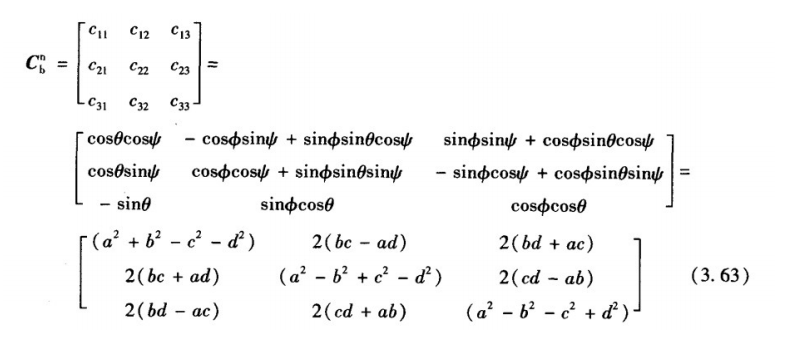由上图可以看出，算法中第二步的时候，直接使用了DCM矩阵的第三行，这点对于初学者可能百思不得其解，但是如果写为矩阵与向量相乘就很清楚了，即vectorA=matrixC*vectorB，在该算法里面，matrixC是图中所示矩阵的转置（因为图中的矩阵为机体坐标转世界坐标，其转置就是世界坐标转机体坐标，这是DCM矩阵的性质之一）；vectorB是标准的重力加速度（0,0,1），使用标准的重力加速度是因为在机体运行过程中，在世界坐标系下（即以人的角度去观察机体坐标系的话），只受到垂直向下（Z轴）的力的作用，这个力就是重力，加速度为9.8m/s/s,而xy轴不受到力的作用，所以数据是0，注意，这里的标准加速度使用归一化之后的值，这样的使用是用深意的，读者将会在第三步体会到；最后vectorA就是我们得到的标准重力加速度在机体坐标系下的映射啦，读者可以在纸上进行上述公式的演算。
3、对机体加速度与标准加速度的映射进行向量的叉乘，得到补偿量，这一步可以说是本算法中最精髓的部分，我们都知道，向量具有点乘和叉乘两种运算，点乘公式为A·B=|A||B|*cosθ，叉乘的公式为A×B=|A||B|*sinθ,其中θ为两个向量之间的夹角，这两个运算的实际的意义读者可以自行查看，这里不再赘述，这里只说在本算法中，A和B是两个单位向量，因此其模为1，所以这样的公式下来就只剩下sinθ了，而在数学上，当θ极小的时候，sinθ是等于θ的，因此这里的公式走下来之后就得到了机体坐标系下实际加速度向量与理想加速度向量之间的差角，下面的算法使用这个差角作为偏差进行PI运算，补偿角速度，这里要特别解释一下，假如我们没有修正角速度，则四元数会因为角速度的漂移而漂移，因此得到的DCM矩阵也会漂移，这时候标准重力加速度在机体坐标系下的映射也会漂移，但是加速度计的数据是长期稳定的，它会与漂移之后的映射不重合，两个向量之间就会产生角度差（由于时间很短，认为是角速度），使用这个角度差矫正角速度数据，就会使抑制角速度长期不稳定，得到准确的四元数。这里建议读者对两个向量的叉乘在纸上进行演算加深印象。
4、PI运算，这里特别强调一下，因为飞行器在飞行过程中机架的震动很容易传到传感器上，加速度又因为短期不稳定，十分容易受到这样震动的影响，变得不稳定，所以在矫正角速度的时候，PI值不易过大，一般P值小于1，I值小于0.01，当然，如果防震措施较好，则可以适当大一些。
5、四元数的微分公式，读者可以自行到网上或者书上找到，这里不再赘述。
展开全文• ## MPU6050姿态解算——Mahony互补滤波

千次阅读 多人点赞 2020-07-18 11:37:43
Long time no see，首先放一张图，黄色的是DMP获取的Pitch角数据，紫红色的是互补滤波解算的Pitch角数据（人为晃动陀螺仪增加了干扰）。 DMP与互补滤波的优缺点分析 DMP 互补滤波 不需要了解姿态解算知识，...
引入
DMP与互补滤波数据对比
Long time no see，首先放一张图，黄色的是DMP获取的Pitch角数据，紫红色的是互补滤波解算的Pitch角数据（人为晃动陀螺仪增加了干扰）。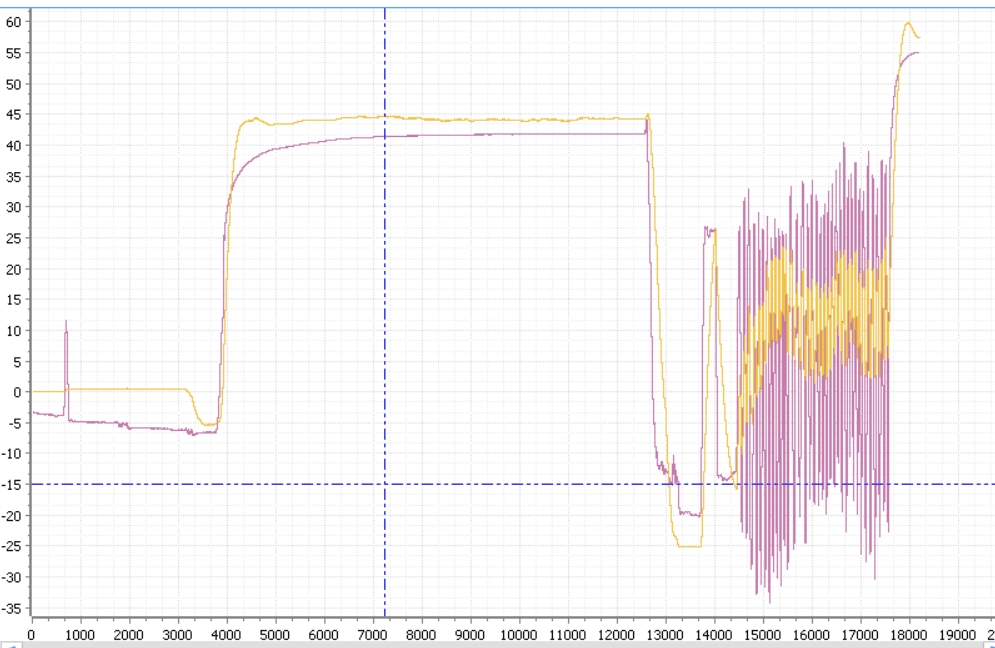DMP与互补滤波的优缺点分析

DMP
互补滤波

不需要了解姿态解算知识，直接调用库函数，操作简单
需要掌握一定的姿态解算知识

解算速度慢，最大只能到200Hz
速度快，而且随着单片机主频提高会更快

真实性差
真实性较好

从上面的曲线图结合我实际的干扰，在我大范围晃动陀螺仪的时候，DMP的角度变化还是很小，感觉是为了滤波而滤波，数据的真实性不可靠。而且在动态曲线观察的时候，互补滤波明显比DMP得到的的数据更快，时效性也🆗。
当然这也不意味着DMP一无是处，在对数据要求没那么高的情况下还是适用的。
接下来就分析一下互补滤波是怎么通过陀螺仪的原始数据解算出较为准确的姿态角儿~。
Mahony互补滤波算法分析
概述
陀螺仪一般为六轴或者九轴构成，六轴就是三轴角速度计加上三轴加速度计，九轴就是在六轴的情况下再加上一个三轴的磁力计，而应用最广泛的MPU6050就是一个六轴陀螺仪。
有一种现象很普遍也包括我自己，就是在没有用过陀螺仪之前，总以为陀螺仪可以直接得到角度数据，实际上并不然，陀螺仪（在下文中就代表MPU6050等六轴陀螺仪）只能得到角速度数据和角速度数据，从而间接得到角度。
可能数理基础不错的小伙伴会想到，对角速度积分不就成了角度吗？没错，确实是这样，但是陀螺仪存在误差，假设陀螺仪有一个0.1°/s的误差，一分钟之后误差就到了6°，一个小时后就是360°，所以原始数据并不是可靠的。
互补滤波就是对误差进行一个补偿，但是并不是用这种积分的方法，而是一个神奇的算法。
欧拉角描述方向余弦矩阵
在用欧拉角描述方向余弦矩阵之前先来了解一下坐标系的变换。注意是坐标系的变换而不是坐标。这篇文章就推导的十分详细
空间中任意坐标的旋转，等效于依次绕三个坐标轴的定轴旋转(不用看图的旋转方向，单纯解释这句话)。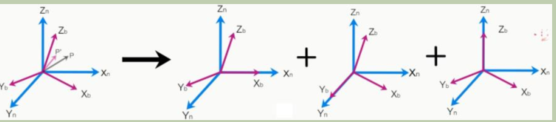以下三个定轴旋转都是在右手坐标系下的正方向旋转：
1. 绕Z轴旋转ψ角，有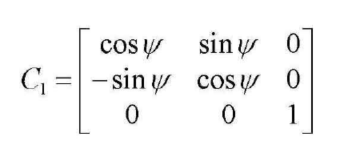2. 绕Y轴旋转θ角，有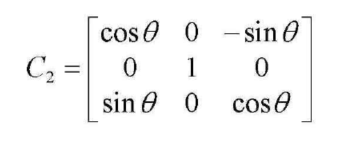3. 绕轴转动γ角，有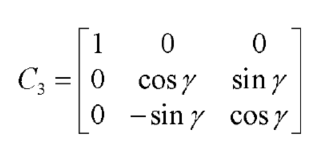4. 整体坐标系转换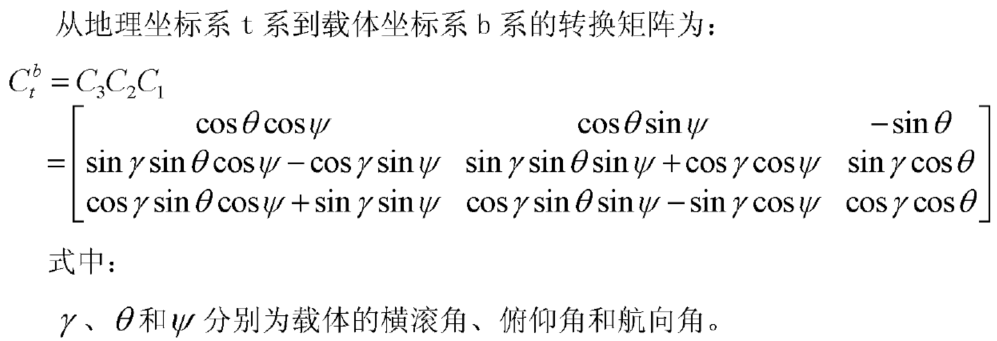本文采用北东地坐标系为地理坐标系，并以地理坐标系作为导航坐标系，所以b系到t系的转换矩阵也是b系到n系的转换矩阵。b到n的转换矩阵被称为姿态矩阵或方向余弦矩阵。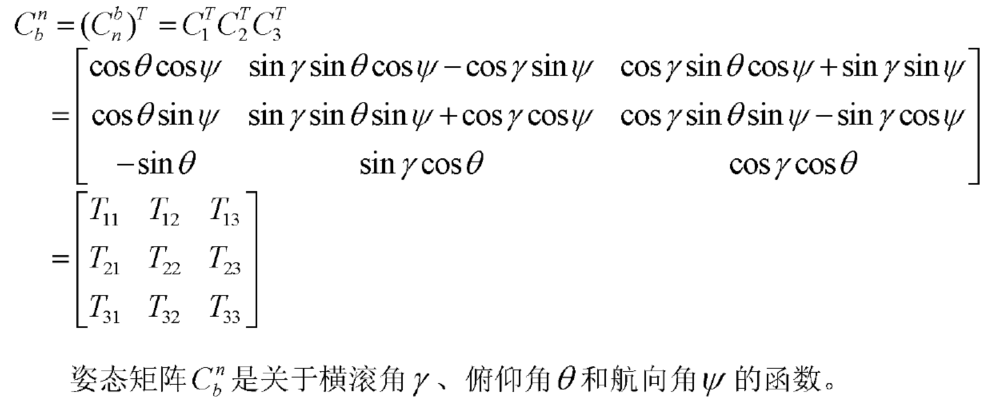由姿态矩阵可以求解出载体的横滚角γ、俯仰角θ和航向角ψ的主值，即：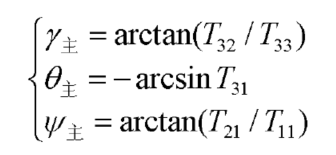这样就解算出了欧拉角，但是用欧拉角描述的方向余弦矩阵伴随着大量的三角运算，对单片机的处理不友好，会影响解算速度，而且会出现一个欧拉角死锁问题点这里，故采用接下来的四元数做解算。
四元数描述方向余弦矩阵
顺口提一句，四元数的发明者就是互补滤波的发明者叫威廉姆·汉密顿，懂为啥要用四元素结算了吧。当然是开个玩笑，四元数也是一种表达姿态的形式，在姿态解算中它避免了大量的三角运算，处理速度快。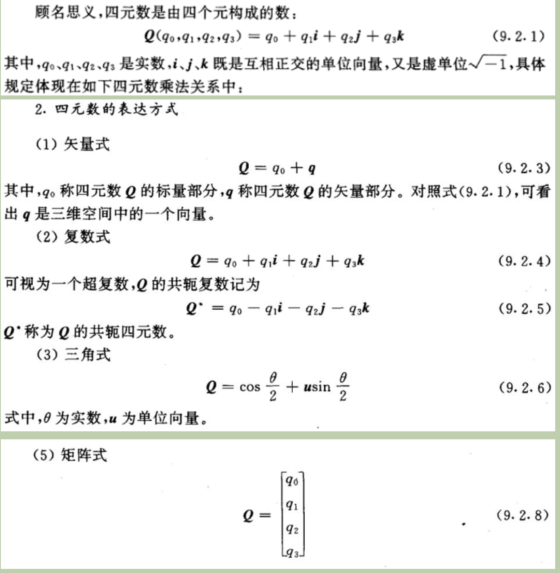用四元数描述上面的用欧拉角描述的姿态矩阵(在阅读开源程序代码的时候一定要注意，可能这个矩阵的不同，那是因为旋转方向和顺序不一样，还要注意看清下标，是哪个系转换到哪个系)：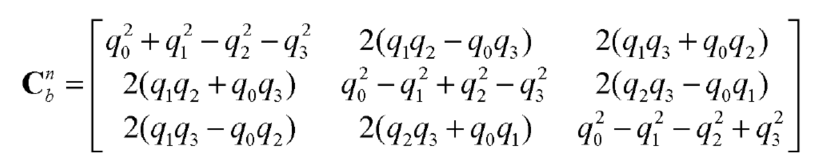由上面欧拉角的主值公式可以得到欧拉角与四元数的关系，这样就通过四元数来表示了方向余弦矩阵和欧拉角。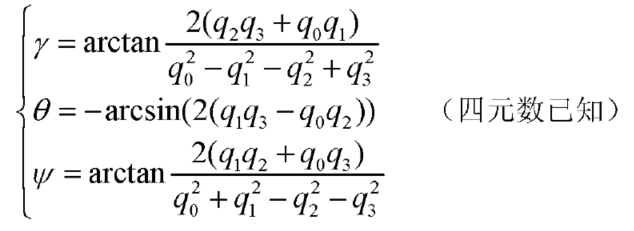求解四元数
把四元数表示的姿态矩阵对时间求微分(其中做左边一列q上面带个小点表示微分，ω就是各轴的角速度)：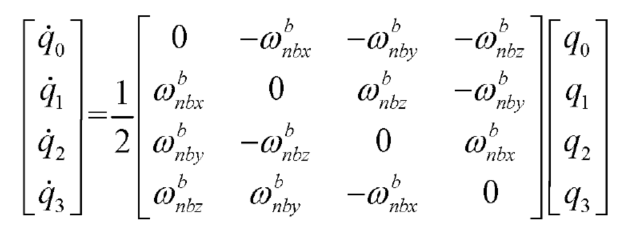然后采用龙格库塔法解微分方程(我还不会推，直接用结论)：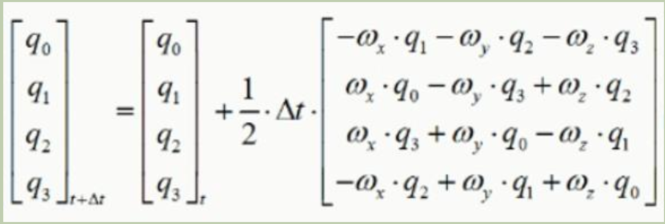下标为t+▲t是当前四元数，右边下标t为上个周期四元数。
传感器数据融合
在概述中我们分析了陀螺仪中角速度测量可能存在一个误差，这个误差在短时间内可以忽略，但是随着时间的累积，误差会越来越大。陀螺仪中的加速度计对高频信号敏感，在振动环境中高频干扰大。
陀螺仪得到的角速度数据短期内可信，长期由于积分误差不可信。加速度计得到的加速度数据，短期内由于高频抖动干扰不可信，长期可信。
拿出我们的中学二年级知识，地球上一切物体的重力加速度都是9.8m/s^2，我们用地理坐标系的重力加速度g，乘以一个从地理坐标系n到机体坐标系b的姿态转换矩阵，可以推导出机体坐标系的理论重力加速度v。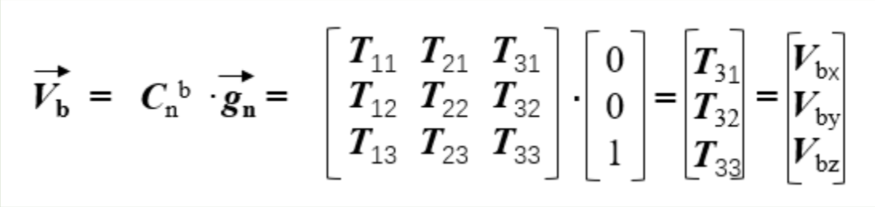误差补偿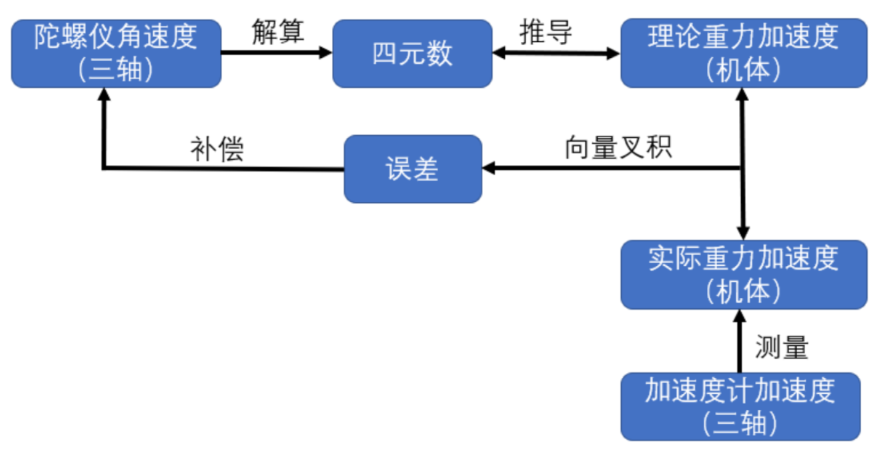由上图可以看出，陀螺仪测出角速度解算出四元数，再由四元素推导出理论重力加速度v，而加速度计测出实际重力加速度a又称gb(b系下的重力加速度)，如果陀螺仪测出的角速度完全可靠，那么实际重力加速度gb应该是等于理论重力加速度vb的，但是很不幸，角速度数据存在误差，vb和gb就不会重合。
灵魂来了
用一个叉积来描述这个误差的大小
几何意义表示：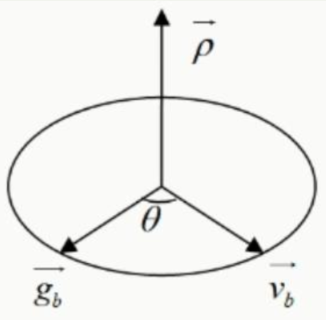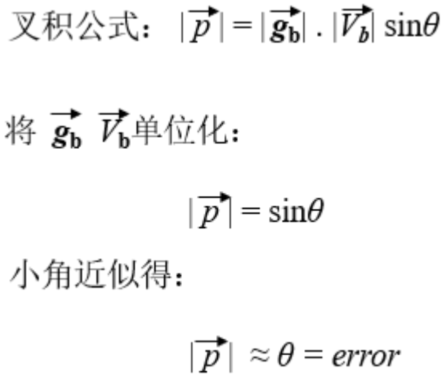叉积公式(两种表达形式，第二种在程序里体现)：

aXb = |a|*|b|*sin θ
对于向量a（x1， y1）， b（x2， y2），叉乘公式为x1 * y2 - x2 * y1

小角近似(sin θ的值与θ的值在θ很小的时侯很相近)：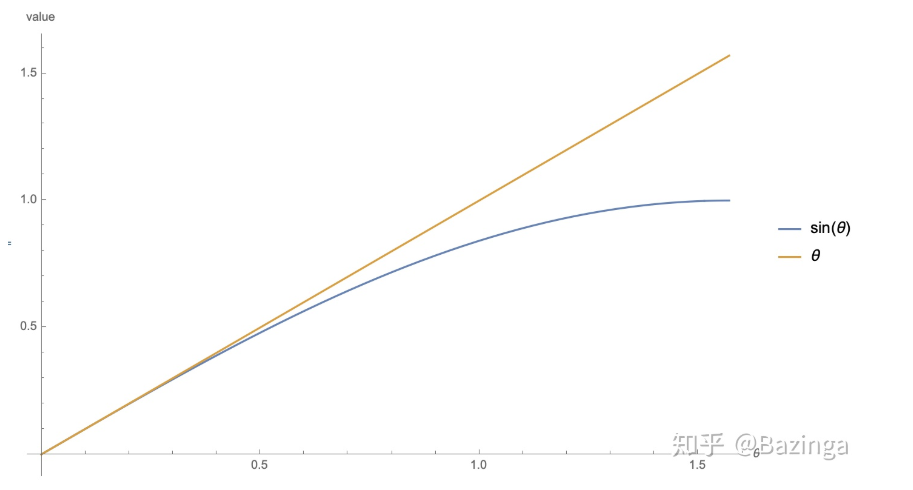在上面得到了理论重力加速度与实际重力加速度的误差erroor，这个error是一个实际的角度误差，我们用加速度计的值间接得出了误差变化的角度相关值，现在借助PID中的PI思想将这个误差补偿，这样就通过加速度计间接的得到了角度误差值(KP=1.5，KI=0.005，是哪篇论文研究过我忘记了，参数可调，按实际来)：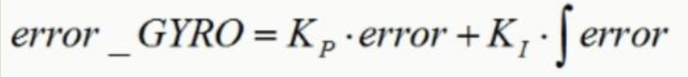偏差角度很小的情况下，我们可以将陀螺仪角速度误差和加速度计求得的角度差看做正比的关系：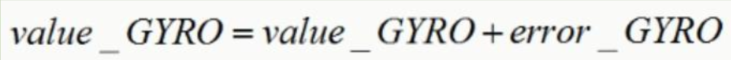得到准确的角速度后就可以用四元数反解出正确可靠的欧拉角了。
STM32程序实例
#include "imu.h"
#include "math.h"
#include "mpu6050.h"
#include "stm32f10x.h"

#define Acc_Gain 0.0001220f			//加速度转换单位(初始化加速度计量程+-4g，由于mpu6050的数据寄存器是16位的，LSBa = 2*4 / 65535.0)
#define Gyro_Gain 0.0609756f		//角速度转换为角度(LSBg = 2000*2 / 65535)
#define Gyro_Gr 0.0010641f			//角速度转换成弧度(3.1415 / 180 * LSBg)
#define G 9.80665f					// m/s^2

extern short ax,ay,az;
extern short gx,gy,gz;

static float invSqrt(float x) 		//快速计算 1/Sqrt(x)
{
float halfx = 0.5f * x;
float y = x;
long i = *(long*)&y;
i = 0x5f3759df - (i>>1);
y = *(float*)&i;
y = y * (1.5f - (halfx * y * y));
return y;
}

void Prepare_Data(void)
{
MPU_Get_Accelerometer(&ax,&ay,&az);			//获取加速度计原始值
MPU_Get_Gyroscope(&gx,&gy,&gz);				//获取角速度原始值

ax = (float)ax * Acc_Gain * G;
ay = (float)ay * Acc_Gain * G;
az = (float)az * Acc_Gain * G;

gx = (float)gx * Gyro_Gr;
gy = (float)gy * Gyro_Gr;
gz = (float)gz * Gyro_Gr;
}

#define Kp 1.50f
#define Ki 0.005f
#define halfT 0.0025f						//计算周期的一半，单位s

extern float Yaw,Pitch,Roll;				//我要给其他文件用所以定义了extern，不用管
float q0 = 1, q1 = 0, q2 = 0, q3 = 0;		//四元数
float exInt = 0, eyInt = 0, ezInt = 0;		//叉积计算误差的累计积分

void Imu_Update(float gx,float gy,float gz,float ax,float ay,float az)
{
u8 i;
float vx,vy,vz;							//实际重力加速度
float ex,ey,ez;							//叉积计算的误差
float norm;

float q0q0 = q0*q0;
float q0q1 = q0*q1;
float q0q2 = q0*q2;
float q0q3 = q0*q3;
float q1q1 = q1*q1;
float q1q2 = q1*q2;
float q1q3 = q1*q3;
float q2q2 = q2*q2;
float q2q3 = q2*q3;
float q3q3 = q3*q3;

if(ax*ay*az == 0)
return;

//加速度计测量的重力方向(机体坐标系)
norm = invSqrt(ax*ax + ay*ay + az*az);			//之前这里写成invSqrt(ax*ax + ay+ay + az*az)是错误的，现在修改过来了
ax = ax * norm;
ay = ay * norm;
az = az * norm;

//四元数推出的实际重力方向(机体坐标系)
vx = 2*(q1q3 - q0q2);
vy = 2*(q0q1 + q2q3);
vz = q0q0 - q1q1 - q2q2 + q3q3;

//叉积误差
ex = (ay*vz - az*vy);
ey = (az*vx - ax*vz);
ez = (ax*vy - ay*vx);

//叉积误差积分为角速度
exInt = exInt + ex * Ki;
eyInt = eyInt + ey * Ki;
ezInt = ezInt + ez * Ki;

//角速度补偿
gx = gx + Kp*ex + exInt;
gy = gy + Kp*ey + eyInt;
gz = gz + Kp*ez + ezInt;

//更新四元数
q0 = q0 + (-q1*gx - q2*gy - q3*gz)*halfT;
q1 = q1 + (q0*gx + q2*gz - q3*gy)*halfT;
q2 = q2 + (q0*gy - q1*gz + q3*gx)*halfT;
q3 = q3 + (q0*gz + q1*gy - q2*gx)*halfT;

//单位化四元数
norm = invSqrt(q0*q0 + q1*q1 + q2*q2 + q3*q3);
q0 = q0 * norm;
q1 = q1 * norm;
q2 = q2 * norm;
q3 = q3 * norm;

//四元数反解欧拉角
Yaw = atan2(2.f * (q1q2 + q0q3), q0q0 + q1q1 - q2q2 - q3q3)* 57.3f;
Pitch = -asin(2.f * (q1q3 - q0q2))* 57.3f;
Roll = atan2(2.f * q2q3 + 2.f * q0q1, q0q0 - q1q1 - q2q2 + q3q3)* 57.3f;
}



展开全文嵌入式 算法 线性代数
• //移植只需改以下参数 /* #define IIC_SCL_Pin GPIO_Pin_6 #define IIC_SDA_Pin GPIO_Pin_7 #define IMU_IIC_GPIO GPIOB #define IMU_IIC_RCC RCC_APB2Periph_GPIOB #define IIC_SDA_In() {GPIOB->CRL&=0X0FFFFFFF...
• 九轴姿态解算，四元数互补滤波法，磁力计数据单独，测试四轴可以起飞
• 九轴传感器姿态解算方法（互补滤波和梯度下降法MATLAB）九轴传感器姿态解算方法（互补滤波和梯度下降法MATLAB）九轴传感器姿态解算方法（互补滤波和梯度下降法MATLAB）九轴传感器姿态解算方法（互补滤波和梯度下降法...
• MPU6050姿态解算STM32代码(互补滤波、卡尔曼滤波)，直接可用
• 关于MPU6050一阶互补滤波方法(从原理到代码实现)1.写在前面最近知道自己不用考研后便花了很多时间来准备机械创新设计大赛，在设计的多功能防台风窗中需要到MPU6050对窗户的姿态进行检测，用来反馈到步进电机控制电机...
关于MPU6050一阶互补滤波方法(从原理到代码实现)1.写在前面最近知道自己不用考研后便花了很多时间来准备机械创新设计大赛，在设计的多功能防台风窗中需要到MPU6050对窗户的姿态进行检测，用来反馈到步进电机控制电机转动(别问为什么不用编码器反馈来控制电机转动，问就是穷，当然也存在本项目用的电源电压低，丢步严重的问题。)在MPU6050传感器中一般读到的原始数据为三轴加速度和三轴角速度，那么如何利用这六个数据进行姿态解算便成为了一个首要的问题。可以利用官方的dmp来解算姿态，但是代码移植是个问题，我用的是恩智浦的RT1064，手头只有MSP430和STM32的dmp库，在采用卡尔曼滤波算法发现收敛太慢的情况下，我决定采用经典的一阶互补滤波算法和简单的自适应一阶互补滤波算法来试试看，也从数学原理到代码彻底的把一阶互补滤波原理捋一遍。2.什么是一阶互补滤波以及为什么要用一阶互补滤波假设我们需要测定一个物理量，这个物理量可以通过两个传感器测得，而且其中一个传感器的测量噪声主要集中在高频段，另一个传感器的噪声主要集中在低频段，那么我们可以构造出两个滤波器，其中一个是低通滤波器，另一个是高通滤波器。然后让这两个传感器测得数据分别通过这两个滤波器再进行加权求和：Y=αx1+(1-α)x2 (1)式(1)中Y即为输出的预测值，α为加权系数，如果这个时候α可变，并且时时刻刻保持估算出的Y的方差最小那么α还有一个名字——卡尔曼增益，此时转化为最佳线性滤波——卡尔曼滤波，只是在卡尔曼滤波算法中x1或者x2有一个是实测的，另一个是通过其他传感器再结合数学模型推测的结果，这和我们MPU6050姿态解算很像，比方说我们要解算俯仰角，那么我们需要知到x轴方向的加速度，绕y轴的角速度，这样我们可以通过：pitch1=asin(ax/g); (2)pitch2 =pitch(0)+ ∫Wydt; (3)来算出两个俯仰角。其中ax为x轴方向加速度，g为重力加速度，ax除以重力加速度再求反正弦函数即为从加速度计中推出的俯仰角1(pitch1)。pitch(0)为初始俯仰角(由第一次测得的pitch1得出)，Wy为y轴角速度，角速度对时间积分再加上初始俯仰角即为从陀螺仪推出的俯仰角2(pitch2)。我们来看看这两个角度是否满足一个噪声集中在高频段，一个噪声集中在低频段：图中黄线代表加速度测得角度，绿线代表真实的角度，可以看出黄线基本跟随绿线，说明没有漂移误差，但是存在较为严重的毛刺信号，说明存在高频噪声；图中蓝线代表陀螺仪的积分，可以看出它几乎没有尖锐的毛刺信号，但是随着积分时间的累加，陀螺仪解算出的角度存在极大的漂移，这个漂移可以看做是一个低频噪声，比如漂移了一个常数值，那么就存在一个很大的频率为0的噪声分量，我们利用matlab的快速傅里叶变换来看看是不是这个情况：clear;clc;x=load('mpu6050.txt');x1=[];for n=0:1131j=6*n+1;x1(n+1)=x(j);endk=0:0.01:11.31;figureplot(k,x1);fs=100;T=1/fs;L=1132;t=(0:L-1)*T;Y=fft(x1,L);P2=abs(Y/L);P1=P2(1:L/2+1);f=fs*(0:(L/2))/L;plot(f,P1);没标横纵坐标单位和图例嗯，坏习惯要改，这里横坐标为频率，单位为Hz，纵坐标为对应频率所占的幅值大小。从图中可以看出在第低频段存在严重的噪声，在获得测试数据时我是以5Hz为频率对传感器进行摆动，当然不可能精确到5Hz,所以我们要获得的数据频率应集中在3-5Hz的频段中，但是由于积分漂移，0hz(常量)-2Hz的干扰1信号占了绝大部分，所以我们应该使用高通滤波器把这些低频信号给滤除。同理可得，我们也可以用快速傅里叶变换来分析加速度计解算出的角度信号的幅频特性，这里就不过多展开了，后面有机会讲卡尔曼滤波算法时再写。得到这两个俯仰角信号的特性后，我们知道它满足互补滤波算法对信号的要求，那么如何设计互补滤波算法呢？具体的实现流程为：3.一阶互补滤波的数学推导一阶低通惯性滤波：LPF=1/(τ*s+1) (4)这是一个低通滤波器，它的截止频率为1/τ，假如我们要让该滤波器的截止频率为3，那么τ为1/3，我们在matlab中用bode图看一看是不是这样的：## 标题num=;den=[1/3 1];sys=tf(num,den);bode(sys);得到：看完了低通滤波，我们还差一个高通滤波，简单：HPF=1-LPF就是高通滤波器：一阶高通滤波：HPF=1-1/(τ*s+1) (5)同样设τ为1/3，来看看bode图：num=;den=[1/3 1];sys=1-tf(num,den);bode(sys);现在再次贴出具体设计方案：我们看上图左边部分，首先忽略掉陀螺仪，B(s)为输出，加速度计输出角度A(s)为输入，得到闭环传递函数为:B(s)/A(s)=1/(TAs+1); (6)同理，以陀螺仪输出角速度G(s)为输入，B(s)为输出，得到闭环传递函数为：B(s)/G(s)=TA/(TAs+1); (7)这里有的同志们就要问了，这不是一阶高通滤波的表达式啊，没关系，因为我们一阶高通滤波器输入的是角度，而这里陀螺仪输出的G(s)为角速度啊，角速度积分就是角度，将(7)式变形为：B(s)=TAs/(TAs+1)*G(s)/s;这样来看就是一个一阶高通滤波器了！到此为止1我们已经知道如何在数学上得到一个一阶互补滤波器了，美汁汁！下面我们来看看代码上如何实现，上来先贴出代码：/*一阶补偿*/float Angle_offset(float acx1,float gyroy2){float hk;hk=-100.0f/(8192-100)*(acx1-100)+100;//MPU_Get_Raw_data(&aacx,&aacy,&aacz,&gyrox,&gyroy,&gyroz);Angle_ax = asin((acx1-hk) /8192);   //去除零点偏移,计算得到角度(弧度)Angle_ax = Angle_ax*180/3.14f;     //弧度转换为度gyroy=-1*(gyroy2+3)/16.4f;//if(abs(gyroy)<1.2)Angle1=0.95f*Angle_ax+0.05f*(Angle1 + gyroy*0.01);/*elseAngle1=0.9f*Angle_ax+0.1f*(Angle1 + gyroy*0.01);*/if(Angle1>=90)Angle1=90;else if(Angle1<=-90)Angle1=-90;pitIsrCnt1=true;return Angle1;}这个函数的输入参数1为x轴加速度，参数2为y轴角速度，其余代码不多解释，我们直奔主题，看最关键的一句：Angle1=0.95fAngle_ax+0.05f(Angle1 + gyroy*0.01);为什么要这样写，怎么从数学方面来进行推导？0.95这个系数怎么得来？(我用的是试凑法，0.95为系数试出来刚好收敛速度快且静态误差较小。)下面我们来简单谈一谈。数学到算法的推导：拉式域方程为：变换到时域并且离散化为：将其变形可得：好了，到这一步我们可以看出来算法与该公式的关系了，前面提到的0.95这个系数其实是T/(T+TA),那么如果我们知道一阶互补滤波每一次迭代时间T，然后又能确定我们需要设定的截止频率1/TA是多少，我们就可以定量计算出这个系数，当然不同的系统肯定会标定出不同的系数。在单片机算力不足或者主频不高的情况下，一阶互补滤波可能是比较好的一个选择，当然，如果算力足够，还是推荐使用卡尔曼滤波，后面有空再写一写基于卡尔曼滤波的姿态解算以及自适应的一阶互补滤波，这篇帖子当做学习心得，后面可以留着复习，以此为记。以上。
展开全文• 姿态解算的数据源可来自于陀螺仪或加速度计，他们分别都可以得到当前姿态，但实际上他们都有各自的传感器性能的优缺点，需要取长补短，进行数据融合才能得到真实的姿态，这种方法简称 互补滤波 算法 传感器特性 ...
飞控算法-姿态解算之互补滤波
姿态解算是通过读取各个传感器的数据进行融合最终得到当前飞行器的姿态，姿态通常用Euler角（Roll-Pitch-Yaw）来表示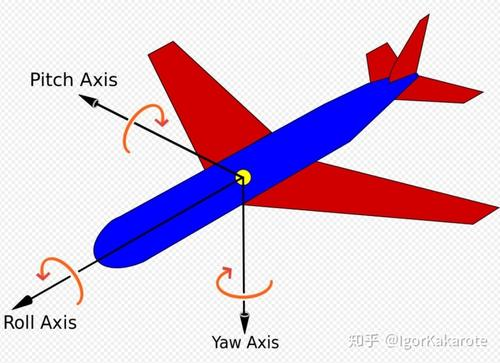姿态解算框架

姿态解算的数据源可来自于陀螺仪或加速度计，他们分别都可以得到当前姿态，但实际上他们都有各自的传感器性能的优缺点，需要取长补短，进行数据融合才能得到真实的姿态，这种方法简称 互补滤波 算法

传感器特性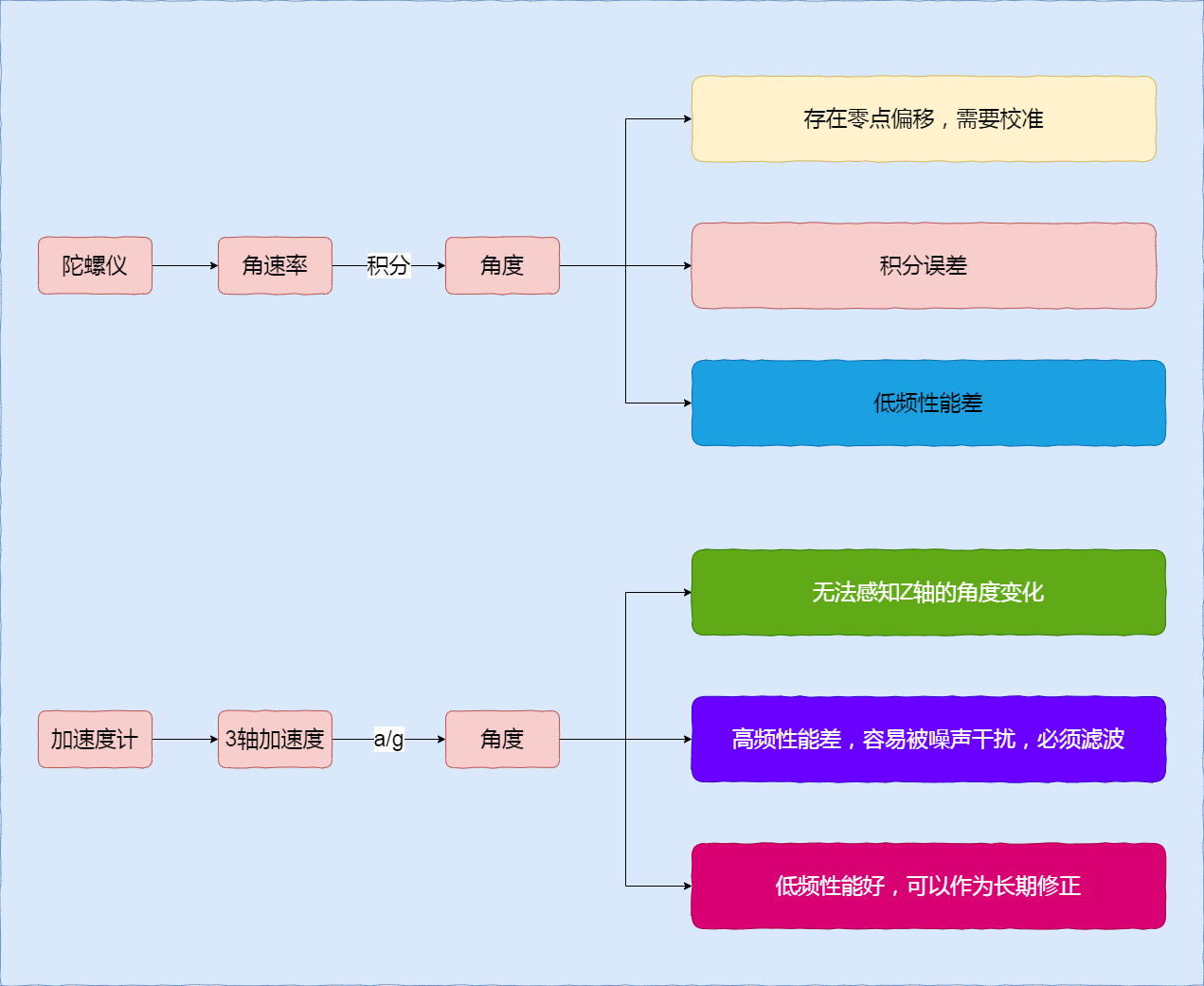互补滤波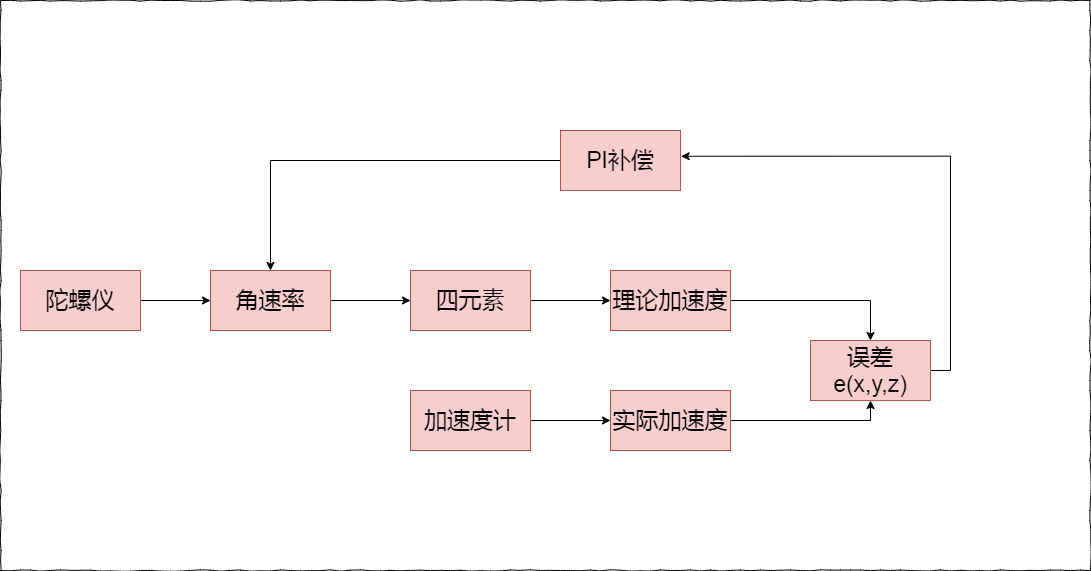姿态解算原理

假设我们现在没有加速度计，只有陀螺仪，那么我们该如何求解姿态呢？

陀螺仪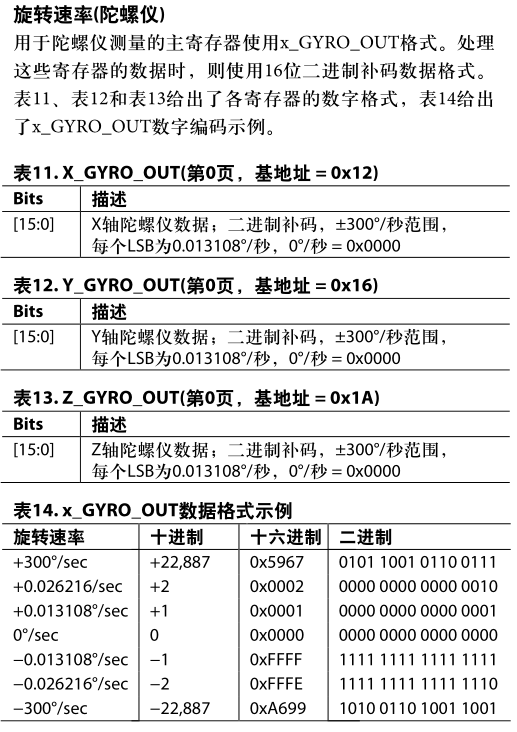这款IMU默认输出为16位的LSB数据，最终得到的角速度为：LSB*Scaler
假如：
X_GYRO_OUT = 22887
Scaler = 0.013108
那么：
g_X = X_GYRO_OUT * Scaler = +300°/sec
也就是说在1秒内旋转了300度，正负根据设备定义的方向，右手定则来决定正负
2、现在得到了角速度，我们可以直接对角速度对时间积分得到当前的角度，这是最简单，最直接的想法，但是通过积分后得到的角度漂移会吓死你，你可以把设备静止在桌面上，观察积分后的角度随直接变化，下面是我直接用角速度积分得到的姿态，用python来绘制的曲线：
代码先送上来：
/*
* 陀螺仪积分求角度
* param1: 原始3轴角速度,单位为x°/s
* param2: 间隔时间,单位为ms
*/
void gyro_int(Vector3f &g, float dt)
{
static Vector3f att(0,0,0);
att.x += g.x * dt * 0.001;
att.y += g.y * dt * 0.001;
att.z += g.z * dt * 0.001;
printf("att is %.3f %.3f %.3f\n", att.x, att.y, att.z);
}

// 主循环代码
void stablize_loop()
{
static uint32_t last_time = 0;
float dt = 0;
if(last_time == 0)
dt = 0;
else
dt = AP_HAL::millis() - last_time;
last_time = AP_HAL::millis();

gyro_int(gyro_data, dt);
}

目前我们只分析X轴的数据：
3、表示姿态有很多种方式，主流的有欧拉角，旋转矩阵，四元数。但都有各自的优缺点，欧拉角最直接但是存在万象锁，旋转矩阵太麻烦耗费资源多，四元数是目前主流的姿态求解的方法。关于四元数的定义和基本概念我这里不做解释了，有兴趣可以自行推导

如何得到四元数

一阶龙格库塔法
公式：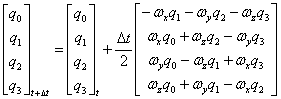q0(t+dt) = q0(t) + 1/2dt * (-Wxq1 - Wyq2 - Wz*q3)
q1(t+dt) = q1(t) + 1/2dt * ( Wxq0 - Wyq3 + Wz*q2)
q2(t+dt) = q2(t) + 1/2dt * ( Wxq3 + Wyq0 - Wz*q1)
q3(t+dt) = q3(t) + 1/2dt * (-Wxq2 + Wyq1 + Wz*q0)

根据公式，我们需要W(x,y,z)，这不就是角速率吗？我们可以直接从陀螺仪里读取到即可
上代码:
/*
* 一阶龙格库塔法求四元数
* 四元数初始值: q0 = 1, q1 = q2 = q3 = 0
* Wx Wy Wz为角速率单位为弧度
* Wx = gx*(PI/180) WY = gy*(PI/180) Wz = gz*(PI/180)
* q0(t+dt) = q0(t) + 1/2dt * (-Wx*q1 - Wy*q2 - Wz*q3)
* q1(t+dt) = q1(t) + 1/2dt * ( Wx*q0 - Wy*q3 + Wz*q2)
* q2(t+dt) = q2(t) + 1/2dt * ( Wx*q3 + Wy*q0 - Wz*q1)
* q3(t+dt) = q3(t) + 1/2dt * (-Wx*q2 + Wy*q1 + Wz*q0)
*/
void gyro_2_quaternion(Vector3f &gyro, float dt)
{
float half_dt = 0.5*dt*0.001; // 单位为秒

float q0_t = 0, q1_t = 0, q2_t = 0, q3_t = 0;

q0_t = half_dt * (-gyro.x * q1 - gyro.y*q2 - gyro.z*q3);
q1_t = half_dt * ( gyro.x * q0 - gyro.y*q3 + gyro.z*q2);
q2_t = half_dt * ( gyro.x * q3 + gyro.y*q0 - gyro.z*q1);
q3_t = half_dt * (-gyro.x * q2 + gyro.y*q1 + gyro.z*q0);

q0 += q0_t;
q1 += q1_t;
q2 += q2_t;
q3 += q3_t;

float normal = sqrt(q0*q0 + q1*q1 + q2*q2 + q3*q3);
q0 /= normal;
q1 /= normal;
q2 /= normal;
q3 /= normal;
//printf("Quaternion is %.3f %.3f %.3f %.3f\n", q0, q1, q2, q3);
}


如何得到欧拉角

旋转矩阵
前面说到，可以通过旋转矩阵来表示姿态，只不过计算量太大，而且存在奇异点，所以没用，但是他在四元数求欧拉角确实很有用的。
首先上旋转矩阵：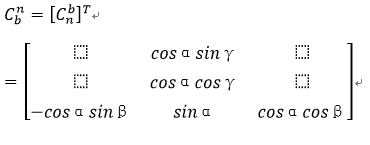旋转方式有很多种，不同的旋转方式得到的矩阵也不一样，要注意！
这里采用东北天坐标系下的Z-X-Y旋转
接着上四元数下的旋转矩阵：
罗德里格旋转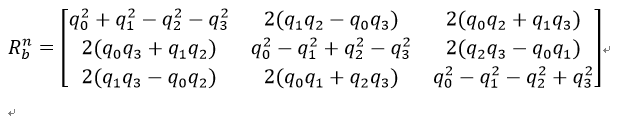接着根据矩阵相等，就可以反解出欧拉角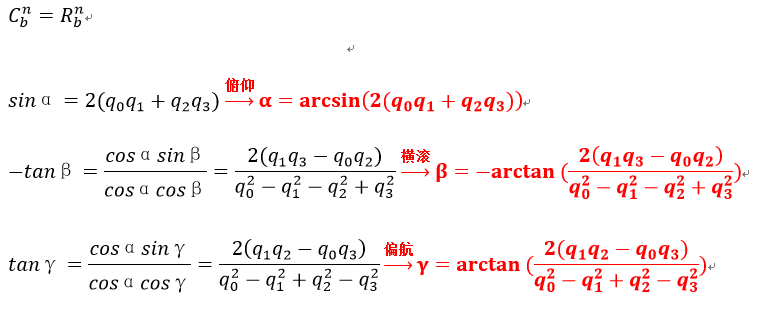送上代码：
/*
* 四元数转欧拉角
*
*
*
*/
Vector3f quaternion_2_euler_angle(float q0, float q1, float q2, float q3)
{
#if 0
// NED北东地坐标系下的Z-Y-X旋转
float roll = atan2f(2.0f*(q0*q1 + q2*q3), (q0*q0 - q1*q1 - q2*q2 + q3*q3));
float pitch = safe_asin(2.0f*(q0*q2 - q1*q3));
float yaw = atan2f(2.0f*(q0*q3 + q1*q2) , (q0*q0 + q1*q1 - q2*q2 - q3*q3));
#else
// ENU东北天坐标系下的Z-X-Y旋转
float roll = -atan2f(2.0f*(q1*q3 - q0*q2), (q0*q0 - q1*q1 - q2*q2 + q3*q3));
float pitch = safe_asin(2.0f*(q0*q1 + q2*q3));
float yaw = atan2f(2.0f*(q1*q2 - q0*q3), (q0*q0 - q1*q1 + q2*q2 - q3*q3));
#endif
return euler_angle;
}

4、问题来了，为啥要用这么复杂的方式转来转去，目的就为了一个，更新四元数，来实现互补滤波

现在我们可以通过四元数来求欧拉角了，但是还是存在一个积分漂移的情况，接下来我们就该我们的加速度计登场了

加速度计

我们可以通过加速度计得到3轴加速度把他和重力加速度求反余弦，就可以得到角度，但是这不是我们需要的！我们需要加速度计是为了获取他的静态性能来更新四元数，补偿陀螺仪
1、得到实际加速度a)
我们从加速度计读取得到的数据是实际的加速度值g，这里注意从传感器里读出的原始加速度值mg，这是一个力的单位，我们一般要把他转换为加速度单位m/s/s，只需要将mg * 9.80665f * 0.001，记得数据要归一化处理哦
    //归一化
acc_data.normalize();

2、得到理论加速度a’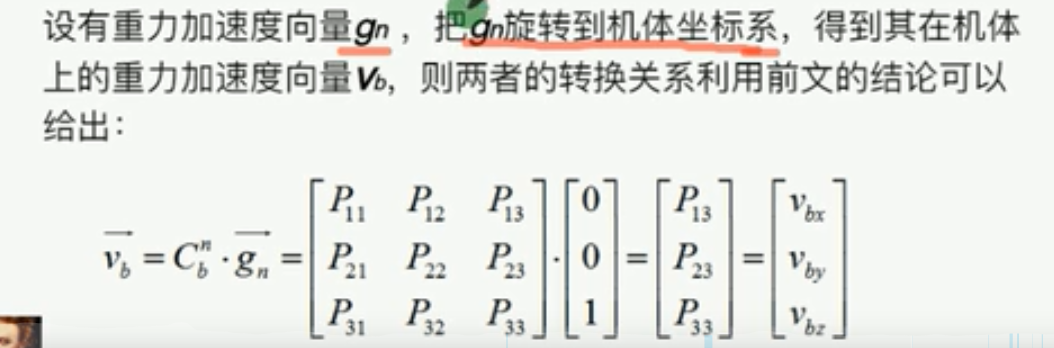我们的四元数又该出场了！
/*
* 四元数得出理论三轴加速度
* ax = 2(q1*q3 - q0*q2)
* ax = 2(q0*q1 + q2*q3)
* az = q0*q0 - q1*q1 - q2*q2 + q3*q3
*/
void quaternion_2_acc(Vector3f &acc, float q0, float q1, float q2, float q3)
{
acc.x = 2*(q1*q3 - q0*q2);
acc.y = 2*(q0*q1 + q2*q3);
acc.z = q0*q0 - q1*q1 - q2*q2 + q3*q3;
}


3、得到误差a(e)
向量叉乘
a(e) = a X a’ = |a| * |a’| * sinr = sinr
小角近似
a(e) = r
得到的误差角为r
上代码：
/*
* 向量叉乘得到误差e
* ex = ay * vz - az * vy
* ey = az * vx - ax * vz
* ez = ax * vy - ay * vx
*/
void vector_cross(Vector3f &a, Vector3f &v, Vector3f &e)
{
e.x = a.y * v.z - a.z * v.y;
e.y = a.z * v.x - a.x * v.z;
e.z = a.x * v.y - a.y * v.x;
}

4、误差得到了，现在该上我们的PI补偿控制器了
这个控制器的作用，就是为了把计算出来的误差补偿到陀螺仪上，使之快速收敛到真实的数据上去，最后得到一个补偿后的陀螺仪数据
上代码:
/*
* 互补滤波算法
* PI补偿控制器u=kp*error+ ki*∫error
*
*/
void complementary_filter(Vector3f &_g, Vector3f &g, Vector3f &e)
{
float Kp = 0.8f;
float Ki = 0.001f;

//比例项
Vector3f g_P(e.x * Kp, e.y * Kp, e.z * Kp);

//积分项
static Vector3f int_e(0, 0, 0);
Vector3f g_I;

g_I.x = int_e.x + e.x * Ki;
g_I.y = int_e.y + e.y * Ki;
g_I.z = int_e.z + e.z * Ki;

_g = g + g_P + g_I;
}

5、现在陀螺仪的数据是补偿后的了，我们可以通过转四元数再反解欧拉角得到补偿后的姿态
全部代码如下：
static float q0 = 1, q1 = 0, q2 = 0, q3 = 0;

/*
* 四元数转欧拉角
*
*
*
*/
Vector3f quaternion_2_euler_angle(float q0, float q1, float q2, float q3)
{
#if 1
// NED北东地坐标系下的Z-Y-X旋转
float roll = atan2f(2.0f*(q0*q1 + q2*q3), (q0*q0 - q1*q1 - q2*q2 + q3*q3));
float pitch = safe_asin(2.0f*(q0*q2 - q1*q3));
float yaw = atan2f(2.0f*(q0*q3 + q1*q2) , (q0*q0 + q1*q1 - q2*q2 - q3*q3));
#else
// ENU东北天坐标系下的Z-X-Y旋转
float roll = -atan2f(2.0f*(q1*q3 - q0*q2), (q0*q0 - q1*q1 - q2*q2 + q3*q3));
float pitch = safe_asin(2.0f*(q0*q1 + q2*q3));
float yaw = atan2f(2.0f*(q1*q2 - q0*q3), (q0*q0 - q1*q1 + q2*q2 - q3*q3));
#endif
return euler_angle;
}

/*
* 一阶龙格库塔法求四元数
* 四元数初始值: q0 = 1, q1 = q2 = q3 = 0
* Wx Wy Wz为角速率单位为弧度
* Wx = gx*(PI/180) WY = gy*(PI/180) Wz = gz*(PI/180)
* q0(t+dt) = q0(t) + 1/2dt * (-Wx*q1 - Wy*q2 - Wz*q3)
* q1(t+dt) = q1(t) + 1/2dt * ( Wx*q0 - Wy*q3 + Wz*q2)
* q2(t+dt) = q2(t) + 1/2dt * ( Wx*q3 + Wy*q0 - Wz*q1)
* q3(t+dt) = q3(t) + 1/2dt * (-Wx*q2 + Wy*q1 + Wz*q0)
*/
void gyro_2_quaternion(Vector3f &gyro, float dt)
{
float half_dt = 0.5*dt*0.001; // 单位为秒

float q0_t = 0, q1_t = 0, q2_t = 0, q3_t = 0;

q0_t = half_dt * (-gyro.x * q1 - gyro.y*q2 - gyro.z*q3);
q1_t = half_dt * ( gyro.x * q0 - gyro.y*q3 + gyro.z*q2);
q2_t = half_dt * ( gyro.x * q3 + gyro.y*q0 - gyro.z*q1);
q3_t = half_dt * (-gyro.x * q2 + gyro.y*q1 + gyro.z*q0);

q0 += q0_t;
q1 += q1_t;
q2 += q2_t;
q3 += q3_t;

float normal = sqrt(q0*q0 + q1*q1 + q2*q2 + q3*q3);
q0 /= normal;
q1 /= normal;
q2 /= normal;
q3 /= normal;
//printf("Quaternion is %.3f %.3f %.3f %.3f\n", q0, q1, q2, q3);
}

/*
* 四元数得出理论三轴加速度
* ax = 2(q1*q3 - q0*q2)
* ax = 2(q0*q1 + q2*q3)
* az = q0*q0 - q1*q1 - q2*q2 + q3*q3
*/
void quaternion_2_acc(Vector3f &acc, float q0, float q1, float q2, float q3)
{
acc.x = 2*(q1*q3 - q0*q2);
acc.y = 2*(q0*q1 + q2*q3);
acc.z = q0*q0 - q1*q1 - q2*q2 + q3*q3;
}

/*
* 向量叉乘得到误差e
* ex = ay * vz - az * vy
* ey = az * vx - ax * vz
* ez = ax * vy - ay * vx
*/
void vector_cross(Vector3f &a, Vector3f &v, Vector3f &e)
{
e.x = a.y * v.z - a.z * v.y;
e.y = a.z * v.x - a.x * v.z;
e.z = a.x * v.y - a.y * v.x;
}

/*
* 互补滤波算法
* PI补偿控制器u=kp*error+ ki*∫error
*
*/
void complementary_filter(Vector3f &_g, Vector3f &g, Vector3f &e)
{
float Kp = 0.8f;
float Ki = 0.001f;

//比例项
Vector3f g_P(e.x * Kp, e.y * Kp, e.z * Kp);

//积分项
static Vector3f int_e(0, 0, 0);
Vector3f g_I;

g_I.x = int_e.x + e.x * Ki;
g_I.y = int_e.y + e.y * Ki;
g_I.z = int_e.z + e.z * Ki;

_g = g + g_P + g_I;
}

void get_gyro(Vector3f &g)
{
uint8_t i, sign;
uint16_t gyro = {0};
float gryo_con;

uint8_t reg = {0};
uint8_t res = {0};
uint8_t pTxFinal = {0x0, 0x83};
_dev->transfer_fullduplex(reg, res, 2);
gyro = res<<8|res;

_dev->transfer_fullduplex(reg, res, 2);
gyro = res<<8|res;

_dev->transfer_fullduplex(reg, res, 2);
gyro = res<<8|res;

_dev->transfer_fullduplex(pTxFinal, res, 2);
gyro = res<<8|res;

for(i = 0; i < 3; i++) {
sign = gyro[i]&0x8000;
if(sign)
else

//printf("gryo_con[%d] [%d].\n", i, gryo_con[i]);
}

g.x = gryo_con;
g.y = gryo_con;
g.z = gryo_con;
}

void get_acc(Vector3f &a)
{
uint8_t i, sign;
uint16_t accel = {0};
float accel_con;

uint8_t reg = {0};
uint8_t res = {0};
uint8_t pTxFinal = {0x0, 0x83};
_dev->transfer_fullduplex(reg, res, 2);
accel = res<<8|res;

_dev->transfer_fullduplex(reg, res, 2);
accel = res<<8|res;

_dev->transfer_fullduplex(reg, res, 2);
accel = res<<8|res;

_dev->transfer_fullduplex(pTxFinal, res, 2);
accel = res<<8|res;

for(i = 0; i < 3; i++) {
sign = accel[i]&0x8000;
if(sign) {
accel_con[i] = (-(~(short )accel[i]+1)) * _adis_chip_info._accel_scale *GRAVITY_MSS/1000.0f;
} else {
accel_con[i] = ((short )accel[i]) * _adis_chip_info._accel_scale *GRAVITY_MSS/1000.0f;
}
//printf("accel_con[%d] [%f].\n", i, accel_con[i]);
}

a.x = accel_con;
a.y = accel_con;
a.z = accel_con;
}

/*
* 陀螺仪积分求角度
*
*/
void gyro_int(Vector3f &g, float dt)
{
static Vector3f att(0,0,0);
att.x += g.x * dt * 0.001;
printf("roll is %.3f\n", att.x);
}

/*
* 姿态矩阵更新
*
*/
void attitude_update()
{
Vector3f gyro_data;
Vector3f acc_data;

get_gyro(gyro_data);
get_acc(acc_data);

static uint32_t last_time = 0;
float dt = 0;
if(last_time == 0)
dt = 0;
else
dt = AP_HAL::millis() - last_time;
last_time = AP_HAL::millis();

#if 0
//直接求角度
//gyro_int(gyro_data, dt);

//四元数求角度
//gyro_2_quaternion(gyro_data, dt);
//Vector3f euler_angle = quaternion_2_euler_angle(q0, q1, q2, q3);
//printf("%.3f,%.3f,%.3f\n", euler_angle.x, euler_angle.y, euler_angle.z);
#else
//实际三轴加速度
//归一化
acc_data.normalize();
//printf("normalize acc x[%.3f] y[%.3f] z[%.3f]\n", acc_data.x, acc_data.y, acc_data.z);

//理论三轴加速度
Vector3f _acc;
quaternion_2_acc(_acc, q0, q1, q2, q3);
//printf("_acc x[%.3f] y[%.3f] z[%.3f]\n", _acc.x, _acc.y, _acc.z);

//理论和实际三轴加速度的叉乘得到误差e
Vector3f error_acc;
vector_cross(acc_data, _acc, error_acc);
//printf("error_acc x[%.3f] y[%.3f] z[%.3f]\n", error_acc.x, error_acc.y, error_acc.z);

Vector3f _gyro;
//PI比例积分补偿陀螺仪的数据
complementary_filter(_gyro, gyro_data, error_acc);

//得到新的四元数
gyro_2_quaternion(_gyro, dt);

//新四元数解算出补偿后的欧拉角
Vector3f euler_angle = quaternion_2_euler_angle(q0, q1, q2, q3);
printf("%.3f,%.3f,%.3f\n", euler_angle.x, euler_angle.y, euler_angle.z);
#endif
}

可以对比补偿前后补偿后的角度数据，发现补偿后没有出现积分漂移，而且在动态性能上也能快的收敛。我们可以调整PI控制器的参数K和i来控制更相信谁的数据！
本次就不上传最后的测试数据了，不是没有测试，而是数据搞丢了，懒得重新搞！


展开全文自动驾驶
• 导航坐标系为东北天（ENU），其与机体坐标系（b）的方向余弦矩阵为CbcC_{b}^{c}Cbc​
• 本人亲测非常好用的MPU6050姿态解算STM32源码(互补滤波)算法，希望帮助大家
• STM32互补滤波源码，MPU6050姿态角解算。详细中文注释
• 共轭梯度和互补滤波的结合姿态解算。可以运用在四旋翼等场合。文档也是以一个例子作为说明。但是如果运用在自己的飞控中还要做一些移植。
• MPU6050姿态解算STM32源码(互补滤波)，MPU6050六轴传感器实验例子，学习MPU6050 六轴传感器(三轴加速度+三轴陀螺仪)的使用.
• 关于利用互补滤波进行姿态解算的文章随便一搜就有很多，就不列出来了。关于卡尔曼的理解和原理，觉得这篇挺通俗易懂的，（二）中还附有matlab代码：卡尔曼滤波 -- 从推导到应用：...
• 消除误差的方法有卡尔曼滤波法、互补滤波法、姿态插值法等。考虑到计算能力和现有的资料，本设计采用互补滤波法，达到的效果也比较好。 算法核心思想：利用加速度计来补偿陀螺仪，希望能最大限度消除误差，进行准确...
• 上次我们讨论了姿态解算基础理论以及几个比较重要的公式的一些推导，...互补滤波算法： 顾名思义，是多组数据结合互补，并进行滤波处理稳定输出，得到姿态的算法。而我们使用的传感器就是加速度计和陀螺仪。加速度计算法 数学 传感器 数据
• 姿态解算之显式互补滤波法（MahonyAHRS） #1 前言 本文以四旋翼为背景，说明一下姿态解算中经典的互补滤波算法，也称为Mahony算法。 文章说明：本文使用的机体系是“右-前-上”坐标系， 导航系是“东-北-天坐标系” ...
• ## Pixhawk-姿态解算-互补滤波

万次阅读 多人点赞 2016-09-13 08:49:03
终于说到了正题，姿态解算这一部分很重要，主要的基础就是惯性导航和多传感器数据融合，很多公司都在招这方面的人才，如百度的无人驾驶在找传感器数据融合，网易的人机交互工程师也在找这方面的人，因为它是信息流的...
• MTI的AHRS解算程序，包含互补滤波的一阶、二阶和卡尔曼滤波程序。
• ## 互补滤波公式

千次阅读 2018-08-29 17:48:02
我的四轴飞行器经验总结(四)--互补滤波法进行姿态解算详解 2017年02月26日 09:00:20 阅读数：3419 消除误差的方法有卡尔曼滤波法、互补滤波法、姿态插值法等。考虑到计算能力和现有的资料，本设计采用互补滤波法...
• Mahony互补滤波器是多旋翼姿态解算中常用的一种简单又高效的方法。 在介绍之前，首先来看一些关于传感器的基础知识： 陀螺仪 用于测量角速度，具有高动态特性，它是一个间接测量角度的器件其中一个关键参数就是...
• 1.目标 求四元数q0、q1、q2、q3； 求解飞行器、机器人的欧拉角pitch、roll、yaw；...3.2 四元数互补滤波 这里不对四元数相关模型和算法的推导讲解，直接粘贴代码： /******************************...算法
• 上一次的博文中（点我打开）介绍了互补滤波法的算法流程和程序实现，但是仅仅只是融合了三轴加速度计和三轴陀螺仪的数据解算出姿态。 由于机体水平时，加速度计无法测量绕 Z 轴的旋转量，即偏航角yaw,并且磁力计也...算法 数据 数学...